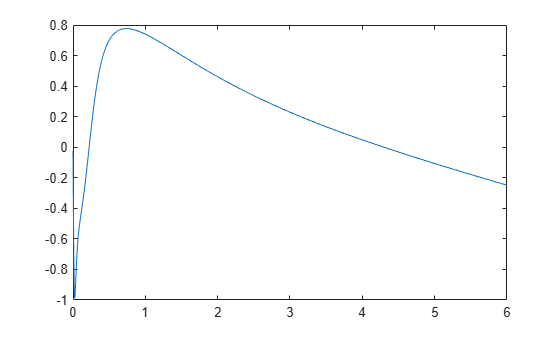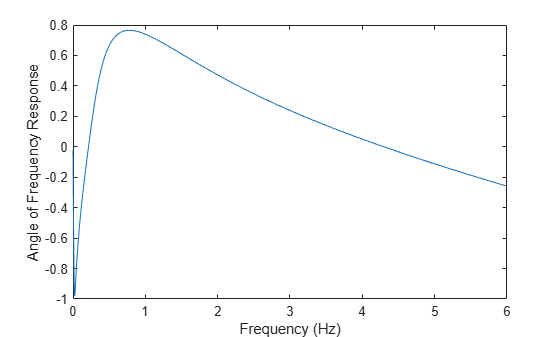# freqresp

Frequency response of rational object and `rationalfit` function object

## Syntax

``[response, outputfreq] = freqresp(fit,inputfreq)``

## Description

example

````[response, outputfreq] = freqresp(fit,inputfreq)` calculates the frequency response, `response` of the `fit` of a `rationalfit` function object or a `rational` object at the specified input frequencies, `inputfreq`.```

## Examples

collapse all

Create a `sparameters` object from a file and use `rfparam` to extract the ${\mathit{S}}_{21}$parameters.

```S = sparameters('passive.s2p'); S21 = rfparam(S,2,1);```

Fit a rational function to the data by using `rationalfit.`

```freq = S.Frequencies; fit_data = rationalfit(freq,S21);```

Compute the frequency response by using the `freqresp` method and plot the magnitude and angle of the frequency response.

```[resp,freq] = freqresp(fit_data,freq); plot(freq/1e9,20*log10(abs(resp)))````plot(freq/1e9,unwrap(angle(resp)))`Perform rational fitting on a S-parameters object by using `rational` object.

```S = sparameters('passive.s2p'); fit_data = rational(S);```

Compute the frequency response by using the `freqresp` function.

```freq = S.Frequencies; [resp,freq] = freqresp(fit_data,freq);```

Plot the magnitude of the frequency response of the ${\mathit{S}}_{21}$data.

```plot(freq/1e9,20*log10(abs(squeeze(resp(2,1,:))))) xlabel('Frequency (Hz)'); ylabel('Magnitude of Frequency Response');```Plot the angle of the frequency response of the ${\mathit{S}}_{21}$data.

```plot(freq/1e9,unwrap(angle(squeeze(resp(2,1,:))))) xlabel('Frequency (Hz)'); ylabel('Angle of Frequency Response');```## Input Arguments

collapse all

N-by-N array, specified as a a `rfmodel.rational` objects returned by `rationalfit` or a `rational` object.

Input frequencies, specified as a vector of positive frequencies in Hz.

Data Types: `double`

## Output Arguments

collapse all

Frequency response values, returned as a vector.

Frequency values same as input frequencies, returned as a vector.